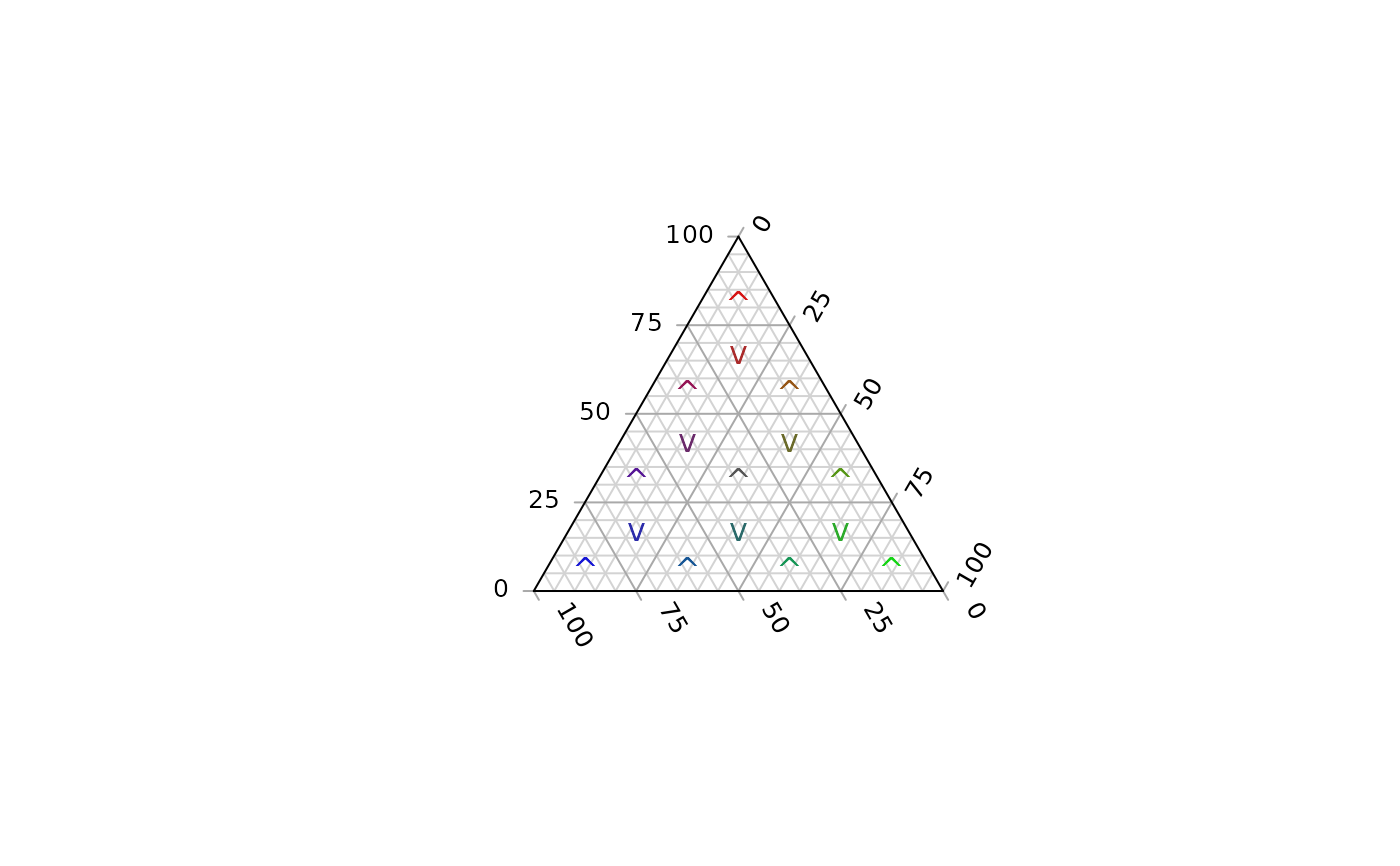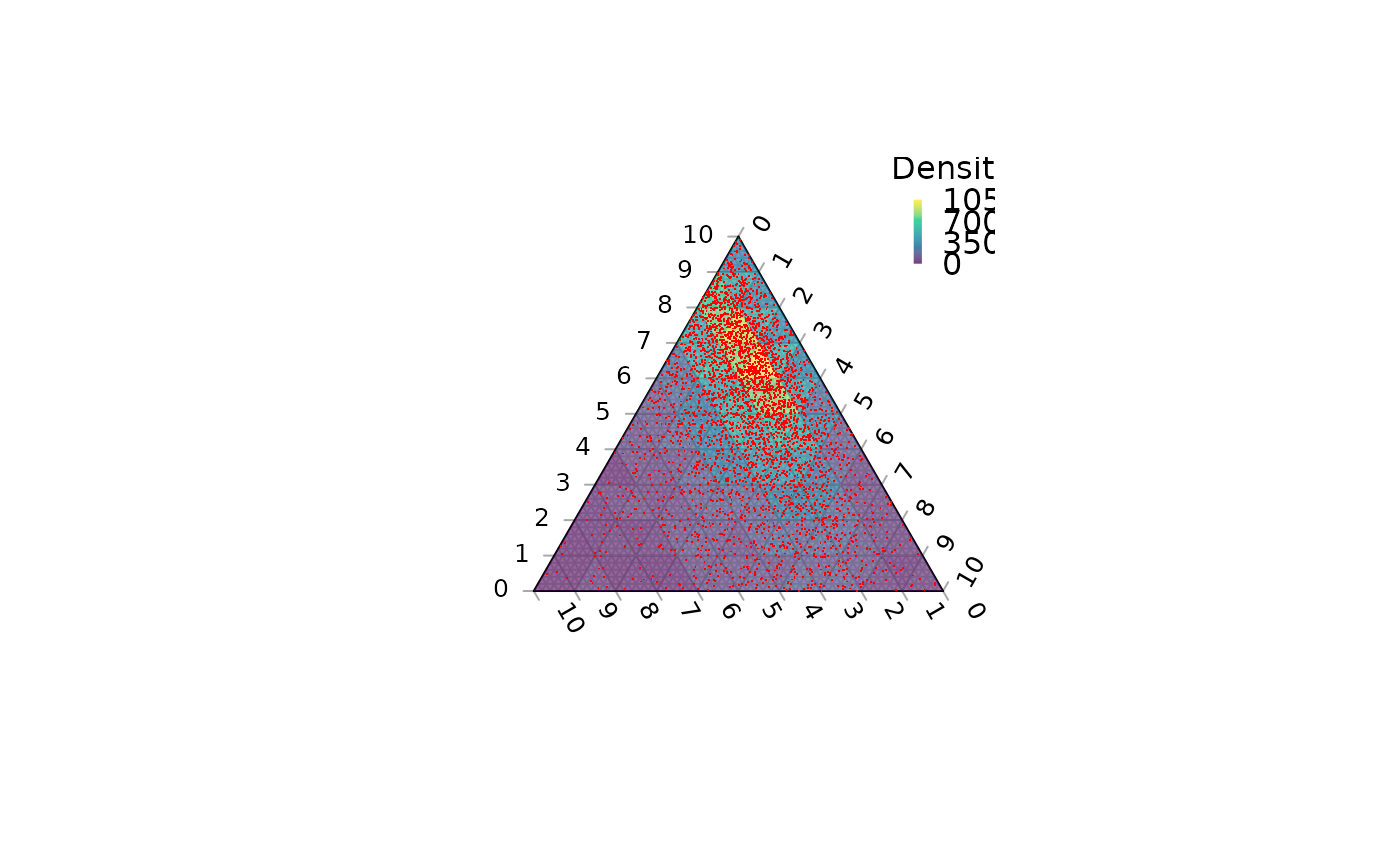Intended to facilitate coloured contour plots with ColourTernary(), TernaryPointValue() evaluates a function at points on a triangular grid; TernaryDensity() calculates the density of points in each grid cell.

TernaryPointValues(
Func,
resolution = 48L,
direction = getOption("ternDirection", 1L),
...
)

TernaryDensity(
coordinates,
resolution = 48L,
direction = getOption("ternDirection", 1L)
)

## Arguments

Func

Function taking vectors of coordinates a, b and c, which returns a numeric vector whose value at each coordinate will be depicted.

resolution

The number of triangles whose base should lie on the longest axis of the triangle. Higher numbers will result in smaller subdivisions and smoother colour gradients, but at a computational cost.

direction

(optional) Integer specifying the direction that the current ternary plot should point: 1, up; 2, right; 3, down; 4, left.

...

Additional parameters to Func().

coordinates

A list, matrix, data.frame or vector in which each element (or row) specifies the three coordinates of a point in ternary space.

## Value

TernaryPointValues() returns a matrix whose rows correspond to:

• x, y: co-ordinates of the centres of smaller triangles

• z: The value of Func(a, b, c), where a, b and c are the ternary coordinates of x and y.

• down: 0 if the triangle concerned points upwards (or right), 1 otherwise

Other contour plotting functions: ColourTernary(), TernaryContour(), TernaryDensityContour()

## Examples

TernaryPointValues(function (a, b, c) a * b * c, resolution = 2)
#>             [,1]       [,2]       [,3]       [,4]
#> x    -0.25000000 0.00000000 0.25000000 0.00000000
#> y     0.14433757 0.28867513 0.14433757 0.57735027
#> z     0.01851852 0.03703704 0.01851852 0.01851852
#> down  0.00000000 1.00000000 0.00000000 0.00000000

TernaryPlot(grid.lines = 4)
cols <- TernaryPointValues(rgb, resolution = 4)
text(as.numeric(cols["x", ]), as.numeric(cols["y", ]),
labels =  ifelse(cols["down", ] == "1", "v", "^"),
col = cols["z", ])TernaryPlot(axis.labels = seq(0, 10, by = 1))

nPoints <- 4000L
coordinates <- cbind(abs(rnorm(nPoints, 2, 3)),
abs(rnorm(nPoints, 1, 1.5)),
abs(rnorm(nPoints, 1, 0.5)))

density <- TernaryDensity(coordinates, resolution = 10L)
ColourTernary(density)
TernaryPoints(coordinates, col = "red", pch = ".")